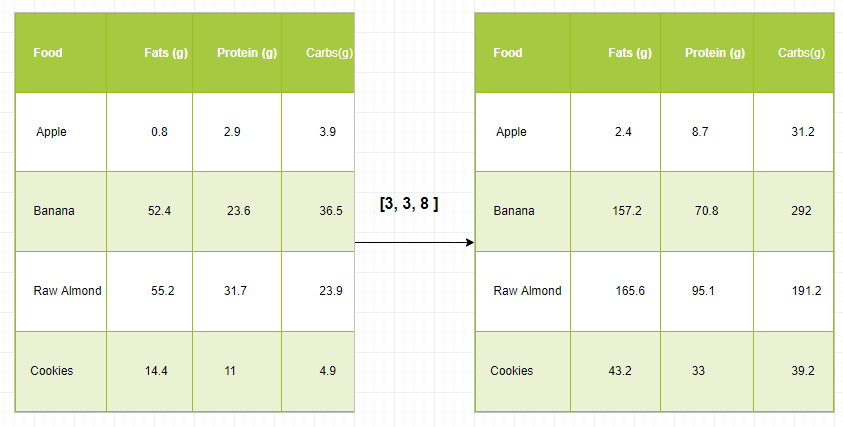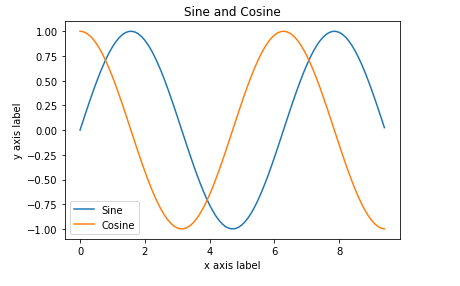Related Articles

# Python | Broadcasting with NumPy Arrays

• Difficulty Level : Expert
• Last Updated : 10 Jun, 2021

The term broadcasting refers to how numpy treats arrays with different Dimension during arithmetic operations which lead to certain constraints, the smaller array is broadcast across the larger array so that they have compatible shapes.
Broadcasting provides a means of vectorizing array operations so that looping occurs in C instead of Python as we know that Numpy implemented in C. It does this without making needless copies of data and which leads to efficient algorithm implementations. There are cases where broadcasting is a bad idea because it leads to inefficient use of memory that slow down the computation.
Example –

## Python3

 `import` `numpy as np` `A ``=` `np.array([``5``, ``7``, ``3``, ``1``])``B ``=` `np.array([``90``, ``50``, ``0``, ``30``])` `# array are compatible because of same Dimension``c ``=` `a ``*` `b``print` `(c)`

Example to get deeper understanding –
Let’s assume that we have a large data set, each datum is a list of parameters. In Numpy we have a 2-D array, where each row is a datum and the number of rows is the size of the data set. Suppose we want to apply some sort of scaling to all these data every parameter gets its own scaling factor or say Every parameter is multiplied by some factor.
Just to have some clear understanding, let’s count calories in foods using a macro-nutrient breakdown. Roughly put, the caloric parts of food are made of fats (9 calories per gram), protein (4 cpg) and carbs (4 cpg). So if we list some foods (our data), and for each food list its macro-nutrient breakdown (parameters), we can then multiply each nutrient by its caloric value (apply scaling) to compute the caloric breakdown of every food item.With this transformation, we can now compute all kinds of useful information. For example, what is the total number of calories present in some food or, given a breakdown of my dinner know how much calories did I get from protein and so on.
Let’s see a naive way of producing this computation with Numpy:

## Python3

 `macros ``=` `array([``  ``[``0.8``, ``2.9``, ``3.9``],``  ``[``52.4``, ``23.6``, ``36.5``],``  ``[``55.2``, ``31.7``, ``23.9``],``  ``[``14.4``, ``11``, ``4.9``]``   ``])` `# Create a new array filled with zeros,``# of the same shape as macros.``result ``=` `zeros_like(macros)` `cal_per_macro ``=` `array([``3``, ``3``, ``8``])` `# Now multiply each row of macros by``# cal_per_macro. In Numpy, `*` is``# element-wise multiplication between two arrays.`` ``for` `i ``in` `range``(macros.shape[``0``]):``     ``result[i, :] ``=` `macros[i, :] ``*` `cal_per_macro` `result`

output:

```array([[   2.4,    8.7,   31.2 ],
[  157.2,   70.8,  292 ],
[   165.6,  95.1,   191.2],
[   43.2,   33,    39.2]])```

### Algorithm:

Inputs: Array A with m dimensions and array B with n dimensions

```p = max(m, n)
if m < p:
left-pad A's shape with 1s until it also has p dimensions

else if n < p:
left-pad B's shape with 1s until it also has p dimensions
result_dims = new list with p elements

for i in p-1 ... 0:
A_dim_i = A.shape[i]
B_dim_i = B.shape[i]
if A_dim_i != 1 and B_dim_i != 1 and A_dim_i != B_dim_i:
else:
# Pick the Array which is having maximum Dimension
result_dims[i] = max(A_dim_i, B_dim_i) ```

1. If the arrays don’t have the same rank then prepend the shape of the lower rank array with 1s until both shapes have the same length.
2. The two arrays are compatible in a dimension if they have the same size in the dimension or if one of the arrays has size 1 in that dimension.
3. The arrays can be broadcast together iff they are compatible with all dimensions.
4. After broadcasting, each array behaves as if it had shape equal to the element-wise maximum of shapes of the two input arrays.
5. In any dimension where one array had size 1 and the other array had size greater than 1, the first array behaves as if it were copied along that dimension.

Example #1: Single Dimension array

## Python3

 `import` `numpy as np``a ``=` `np.array([``17``, ``11``, ``19``]) ``# 1x3 Dimension array``print``(a)``b ``=` `3` `print``(b)` `# Broadcasting happened because of``# miss match in array Dimension.``c ``=` `a ``+` `b``print``(c)`

Output:

```[17 11 19]
3
[20 14 22]```

Example 2: Two Dimensional Array

## Python3

 `import` `numpy as np``A ``=` `np.array([[``11``, ``22``, ``33``], [``10``, ``20``, ``30``]])``print``(A)` `b ``=` `4``print``(b)` `C ``=` `A ``+` `b``print``(C)`

Output:

```[[11 22 33]
[10 20 30]]
4
[[15 26 37]
[14 24 34]]```

Example 3:

## Python3

 `import` `numpy as np` `v ``=` `np.array([``12``, ``24``, ``36``]) ``w ``=` `np.array([``45``, ``55``])   ` `# To compute an outer product we first``# reshape v to a column vector of shape 3x1``# then broadcast it against w to yield an output``# of shape 3x2 which is the outer product of v and w``print``(np.reshape(v, (``3``, ``1``)) ``*` `w)` `X ``=` `np.array([[``12``, ``22``, ``33``], [``45``, ``55``, ``66``]])` `# x has shape  2x3 and v has shape (3, )``# so they broadcast to 2x3,``print``(X ``+` `v)` `# Add a vector to each column of a matrix X has``# shape 2x3 and w has shape (2, ) If we transpose X``# then it has shape 3x2 and can be broadcast against w``# to yield a result of shape 3x2.` `# Transposing this yields the final result``# of shape  2x3 which is the matrix.``print``((x.T ``+` `w).T)` `# Another solution is to reshape w to be a column``# vector of shape 2X1 we can then broadcast it``# directly against X to produce the same output.``print``(x ``+` `np.reshape(w, (``2``, ``1``)))` `# Multiply a matrix by a constant, X has shape  2x3.``# Numpy treats scalars as arrays of shape();``# these can be broadcast together to shape 2x3.``print``(x ``*` `2``)`

Output:

```[[ 4  5]
[ 8 10]
[12 15]]

[[2 4 6]
[5 7 9]]

[[ 5  6  7]
[ 9 10 11]]

[[ 5  6  7]
[ 9 10 11]]

[[ 2  4  6]
[ 8 10 12]]```

Plotting a two-dimensional function –
Broadcasting is also frequently used in displaying images based on two-dimensional functions. If we want to define a function z=f(x, y).
Example:

## Python3

 `import` `numpy as np``import` `matplotlib.pyplot as plt` `# Computes x and y coordinates for``# points on sine and cosine curves``x ``=` `np.arange(``0``, ``3` `*` `np.pi, ``0.1``)``y_sin ``=` `np.sin(x)``y_cos ``=` `np.cos(x)` `# Plot the points using matplotlib``plt.plot(x, y_sin)``plt.plot(x, y_cos)``plt.xlabel(``'x axis label'``)``plt.ylabel(``'y axis label'``)``plt.title(``'Sine and Cosine'``)``plt.legend([``'Sine'``, ``'Cosine'``])` `plt.show()`

Output:Attention geek! Strengthen your foundations with the Python Programming Foundation Course and learn the basics.

To begin with, your interview preparations Enhance your Data Structures concepts with the Python DS Course. And to begin with your Machine Learning Journey, join the Machine Learning – Basic Level Course

My Personal Notes arrow_drop_up